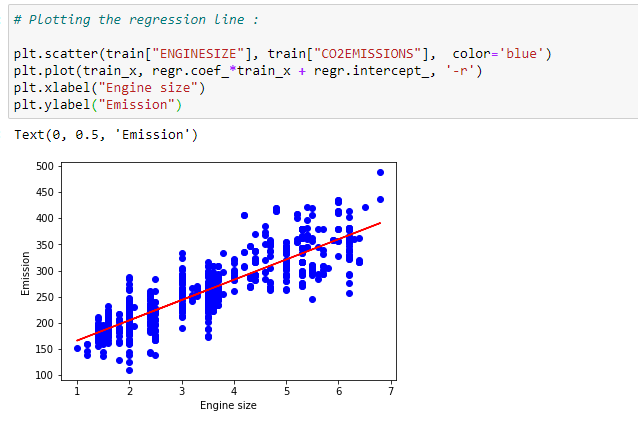# Simple Linear Regression Implementation

Original article was published on Artificial Intelligence on Medium# Simple Linear Regression Implementation

In the last article, we saw how to implement simple linear regression from scratch,but that was a long process, right? We can do the same calculation using scikit-learn in just few lines. Exciting!!!

Well what are we waiting for? Let’s dig in!!

(1) Import the required libraries :

(2) Read the data file :

(3) Select useful features from data :

(4) Plot out data on scatter plot :

(5) Divide data into training and testing data :

(6) Use scikit-learn to find Intercept and Slope :

(7) Plot the regression line :

(8) Predict the value of co2-emission :

Here you can see that we get the exact same value as our previous article!

(9) Check model accuracy :